# SPSS AMOS Serial Mediation Analysis### Serial Mediation Analysis with Multiple Variables in SPSS AMOS

The Focus of the video tutorial is guiding how to analyze a serial mediation model with multiple mediators in SPSS AMOS.

## Introduction to Serial Mediation

• Serial mediation, also called chain mediation, is where the influence of the independent variable flows through multiple mediators before impacting the dependent variable.
• Serial mediation often takes place where the first mediator will have a direct relationship with a second mediator before ultimately having a relationship to the final dependent variable.
• With this type of mediation, you have to account for the indirect effect across multiple constructs.
• To test this type of mediation, we are going to use the estimands function again.

## Step 1: Name the Paths

• In this revised model, the influence or effect of Organizational Learning (OL) is proposed to flow through Collaborative Culture (CC) and Organizational Commitment (OC) to Organizational Performance (OP).
• Put another way, the indirect effect of Organizational Learning (OL) to Organizational Performance (OP) flows through both constructs of Collaborative Culture (CC) and Organizational Commitment (OC).

Next, we need to label the arrows (parameters) between each construct.

• a_path = The path from the independent variable to the first mediator (OL to CC)
• b_path = The path to the ultimate dependent variable from the second mediator (OC to OP).
• d_path = The path between the two mediators (CC to OC).
• c_path = The direct effect from OL to OP.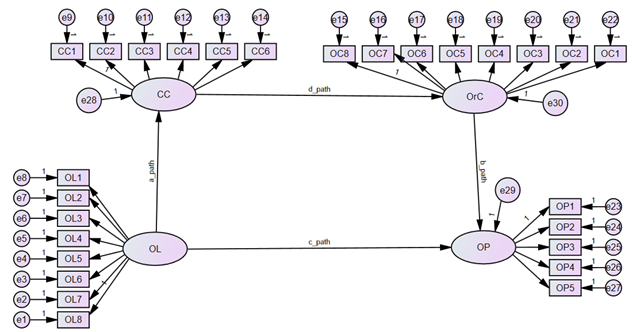## Step 2: Define Estimand

• Now that we have uniquely labeled the parameters, we need to use the estimand function by defining a new function.
• To calculate the indirect effect in serial mediation, you need to multiply the intermediating relationships together.
• Specifically, you will multiply the regression coefficient for each intervening relationship.
• In our example, we are going to multiply the “a_Path” times the “d_Path” times the “b_Path”. This will give us the serial indirect effect. In the estimands function,
• let’s call our indirect test “SM”. In the syntax, we will specify the formula for the indirect relationship. After doing this, we make sure to check for syntax errors, and then we can save and exit the pop-up window.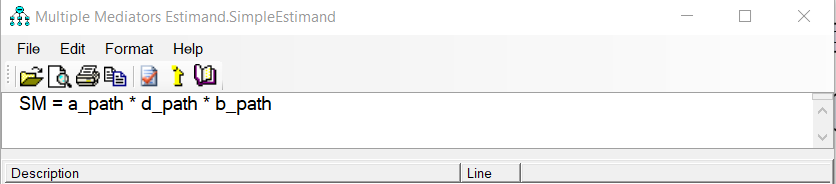## Step 3: Analysis Properties

• To determine if the indirect effect of OL to OP is significant, we need to request from AMOS the indirect, direct, and total effects in the output. This will give all possible indirect effects in the model.
• To do this, select the Analysis Properties button , and when the Analysis Properties pop-up window appears, go to the Output tab at the top. On that tab, you will see at the top right an option for “Indirect, direct, and total effects”. Select this option.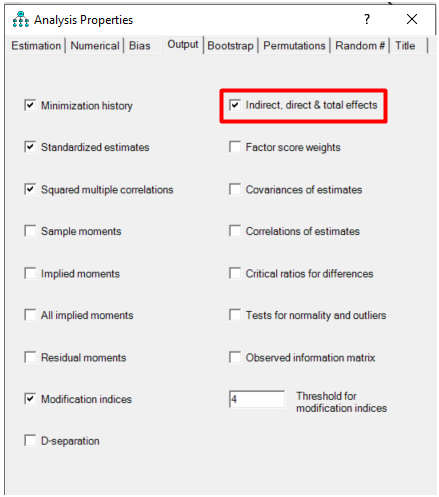• Next, we need to request a bootstrap analysis in AMOS. To do this, go to the bootstrap tab at the top of the Analysis Properties window. On that tab will be a checkbox called “Perform bootstrap”; click that box. AMOS will initially give you a default number of 200 samples. This is way too small. Change the number of samples to 5,000.
• You will also need to select the “Bias-corrected confidence intervals” checkbox. AMOS will default a 90% confidence interval, but significance in most research is at the .05 level, so you need to change this to a 95% confidence level.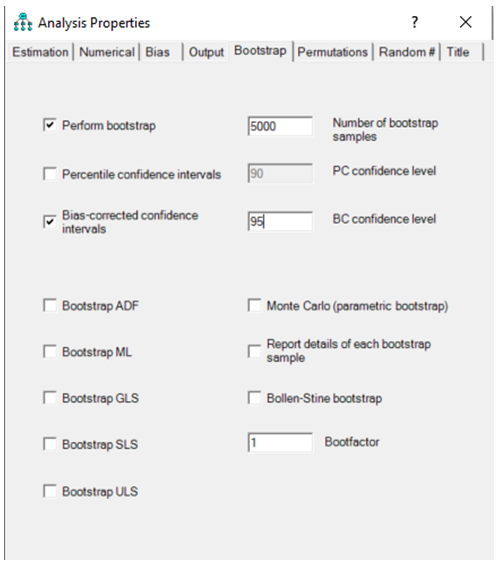Next, Calculate the Estimates

## Analyzing the Results - Step 1

• The results of the indirect effect through both intervening variables to OP was .192, and it is found significant (p = .002).
• We initially can determine that the serial mediation is significant, but we need to examine the direct effects to determine the type of mediation that is present. The direct effect (c_Path) is significant with a p value < .001.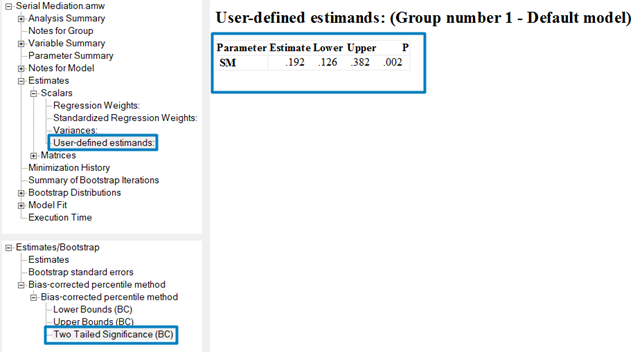• These results show that both the indirect effect and the direct effect are significant. This means partial mediation is present with this serial mediation test.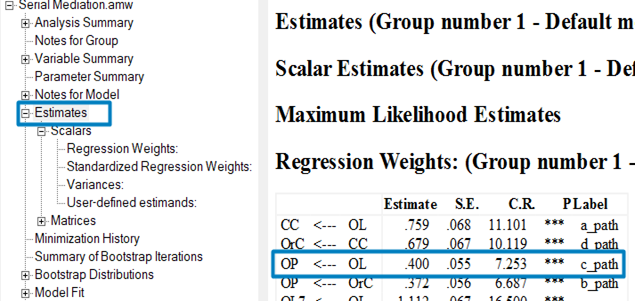• If you have three or mediators, the process would be exactly the same.
• You would label all the parameters and then get the product of all the relationships from the independent to a dependent variable through the mediators. Using the estimands function will make this a relatively easy process.

## Analyzing the Results - Indirect Effects

• Since the indirect effect from OL to OP could go only through the two specified intervening constructs, we should be able to get the same results from the “indirect effects” output that AMOS initially gives us.
• In the output, let’s go to the Estimates tab, “Matrices” option, and then down to the Indirect Effects.
• The indirect effect of OL to OP is .192, the same as the estimands function we ran earlier.
• You will see that AMOS gives us all possible indirect effects.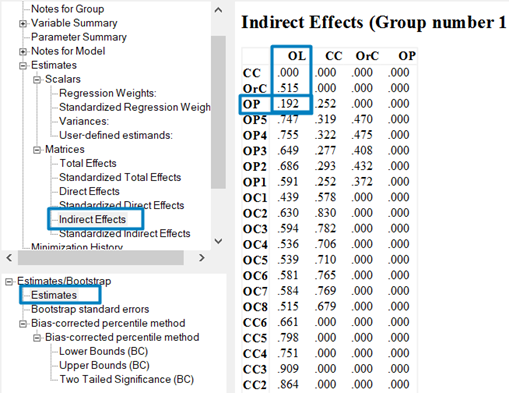Next,

• If we go to the Bias-corrected percentile method from these indirect effects where the bootstrap analysis is presented, you get the same confidence interval and p-value as the one listed in the estimands output.
• Remember, these results are similar to User-defined estimands, only because the indirect effect is going through the only two intervening variables in the model.
• In this example, the indirect effect from OL to OP has no other possible indirect effect but through the two mediators.